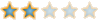# Quickly multiply numbers close to multiples of 10##Quickly multiply numbers close to multiples of 10

The following is a five minute video providing a smarter way of multiplying numbers close to multiples of 10. Testing the new method versus the speed of a TI-83 calculator, you'll find it much faster.

THE BASIC PRINCIPLE:
(10+X)(10+Y)= 100+ 10X+ 10Y+XY= 10(10+X+Y)+XY

In other word, add the units digit of the second number (Y) to the first number (10+X). This will serve as your hundreds and tens digit (that's why it is multiplied by 10 above.) Add the product of the units digit (XY) to 10(10+X+Y.)

For example:
12*13

First it is 10(12+3)= 150
Then add 2*3=6 for,
150+6= 156

This will be highlighted in the video and make things seem easier.

##Re: Quickly multiply numbers close to multiples of 10

That's cool Archis

i have a question though - how did you get a youtube video onto the forum?Steve
The Cool CubePosts : 85
Points : 4
Reputation : 4
Join date : 2008-10-07
Age : 22
Location : ...here##Great Video!

I was wondering if this could work with numbers like, say, 24 and 25. How would you do it and would this work?

BCase
Novice PosterPosts : 14
Points : 2
Reputation : 1
Join date : 2009-02-13
Location : The Land of the Pharaohs##Re: Quickly multiply numbers close to multiples of 10

@BCase: Excellent question you pose

This though only works for base 10. As in it will only work for numbers close to 10, 100, 1000...

Click below for run through of similiar problem except with numbers closer to 100.
Spoiler:
For example:
125*102

Similar steps
(125+2)(100)= 12700
25*2= 50

Note how I multiplied by 100 for the first step, because 100 is the closest base 10 to both numbers.

So, sorry, this same method won't work for 24*25. Though if you want to learn how to solve 24*25, pm me and I'll tell you how.##Re: Quickly multiply numbers close to multiples of 10